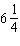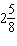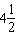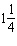Name:    Math 10W Unit 3 Practice Test #4

Multiple Choice
Identify the choice that best completes the statement or answers the question.

1.

What is the perimeter of a square garden with a side length of 3.2 m?
 a. 12.8 m c. 10.2 m b. 9.2 m d. 6.4 m

2.

How much wood do you need to form a triangular garden frame if one side of the frame has a length of 11 ft, and the other two sides are 2 feet longer than the first side?
 a. 36 ft c. 35 ft b. 33 ft d. 37 ft

3.

How many inches is 7'4"?
 a. 55" c. 132" b. 86" d. 88"

4.

Which of the following are the most appropriate imperial units to use to measure the width of a textbook?
 a. centimetres c. feet b. millimetres d. inches

5.

A carpenter measures the perimeter of a room to be 56'".  He needs to buy baseboard to finish the room but the store only sells it by the full metre. How many metres of baseboard will he need to buy?
 a. 2 m c. 18 m b. 174 m d. 1732 m

6.

A square-based pyramid has a base length of 10 feet, a height of 45 inches, and a slant length of 3 yards. What is the surface area, including the base?
 a. 175 ft2 c. 280 ft2 b. 337.5 ft2 d. 200 ft2

7.

Which of these shapes has the largest surface area?
• a cylinder with a height ofinches and a radius ofinches; or
• a rectangular prism with a length of 5 inches, a width ofinches, and a height of 4 inches; or
• a cone with a slanted side ofinches and a radius ofinches; or
• a square-based pyramid with a base length ofinches and a slant length ofinches.
 a. cylinder c. cone b. rectangular prism d. square-based pyramid

8.

Juan fills a 8 L bucket with a container that holds 2 pints. How many times will he have to fill up the container to fill the bucket?
 a. 8 times c. 17 times b. 9 times d. 10 times

9.

Find the volume of the rectangular prism below, in in3.a. 36.5 in3 c. 234.7 in3 b. 143.6 in3 d. 39.3 in3

10.

A garden has an area of 24 yd2. It is covered in topsoil that is 3 in deep. What is the volume of topsoil used, in yd3?
 a. 72 yd3 c. 6 yd3 b. 2 yd3 d. 24 yd3

1.

Yuna is replacing some plumbing pipes. She needs 3 sections of pipe: one that is' long, one that is' long, and one that is' long. How much pipe does she need if she loses" every time she makes a cut? She can only purchase the pipe in full feet.

2.

Charlie drove for 3 hours at 80 km/h.

a)       How far did he travel in kilometres?

b)       How far did he travel in miles? Calculate the distance to the nearest mile.

3.

Sandy is painting the living room in her house. The room measures 18 feet long by 11 feet wide by 8 feet high. She will only paint the walls and not the floor or ceiling. What is the total area Sandy will paint?

4.

A rectangular prism measures 120 cm by 104 cm by 57 cm. Calculate its surface area in square inches. Round your answer to 2 decimal places.

5.

A recipe calls forcups of water. What is this in mL?